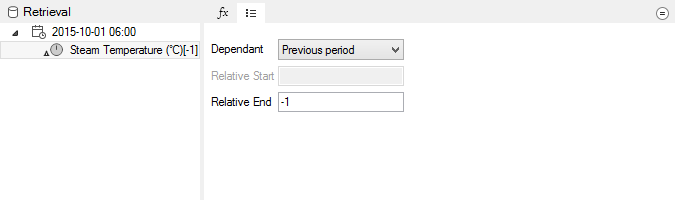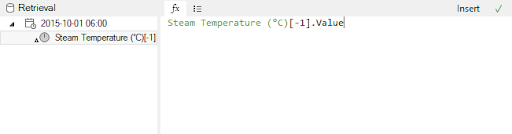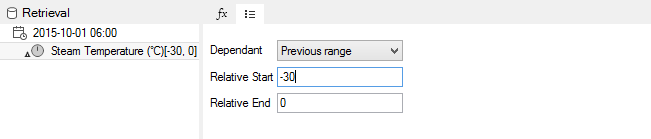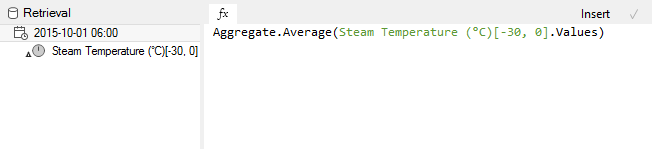Follow

# What if I want to perform a Calculation over a range of Time Periods?

This article will be updated in the near future! In the meanwhile, if the following information is insufficient, contact support@flow-software.com.

For Calculated Measures, Flow allows calculations to be configured based on a measure’s previous period’s value, or even a previous range of values.

### Relative Period

When selecting a dependent measure in a measure calculation, select the “Properties” tab.  Set the “Dependent” property to “Previous period” and the “Relative End” to -1.Flow will now use the previous period of this measure in your calculation expression.### Relative Range

Set the “Dependent” property to “Previous range”, “Relative Start” to -30 and the “Relative End” to 0.Flow will now use an array of values in your calculation expression.  You will need to use this array in a Built-In or User Defined Function.This Relative Range calculation is useful for “moving window” calculations (e.g. moving average, moving sum, etc.)